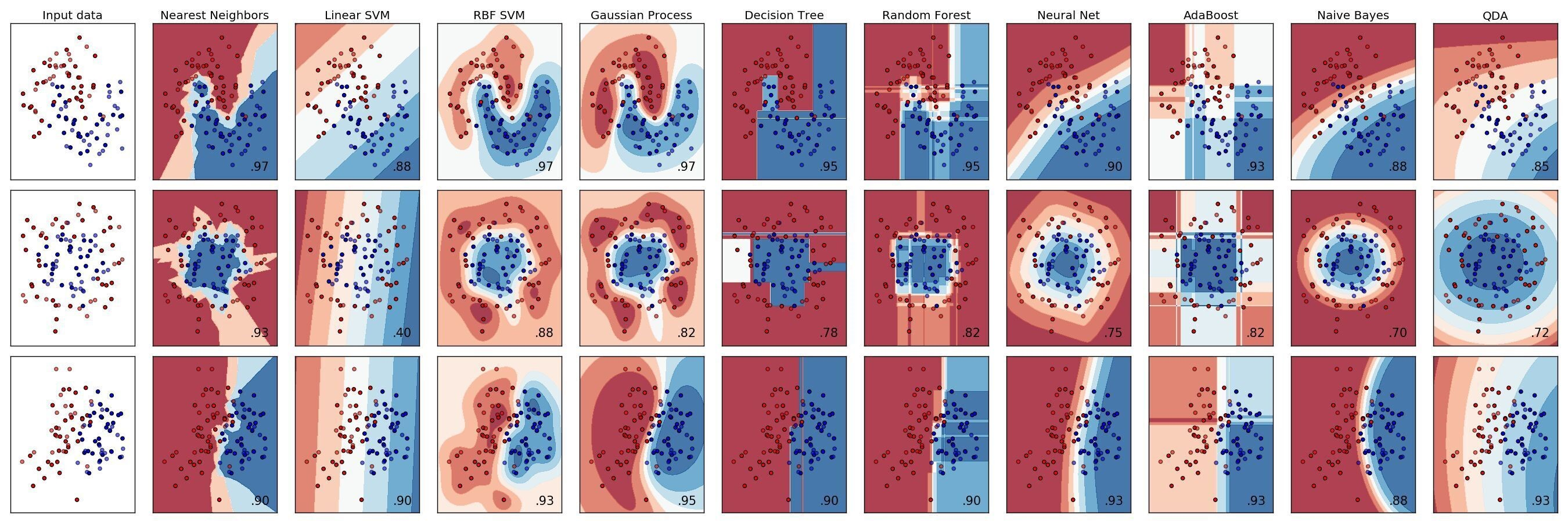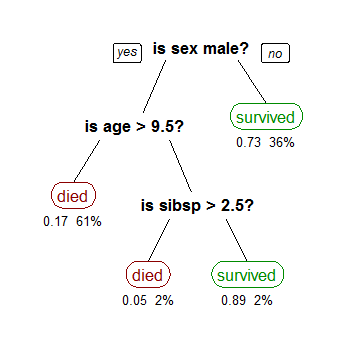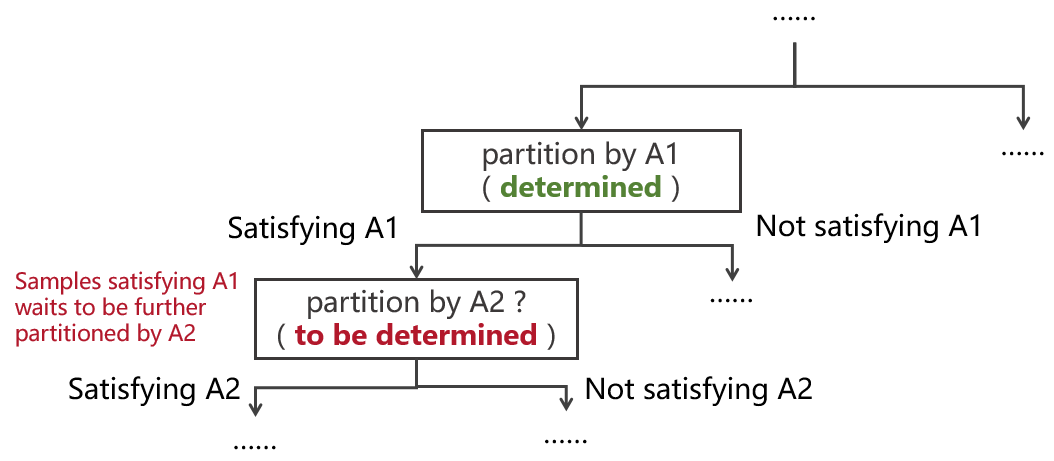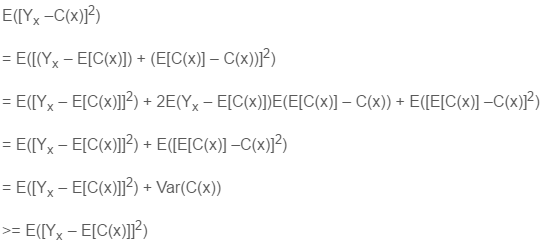Stock selection with classification tree based algorithms (Part I)

Chuan Shi   2016-11-09 本文章911阅读

1

Introduction

The marriage between machine learning and investment populates stock selection using artificial intelligence. In the context of machine learing, stock picking is a typical classification problem. Various financial and technical factors are viewed as features of stocks, and the returns of the assets are used to determine the labels they possess.

Stock selection with machine learning belongs to the domain of supervised learning. It separates good and bad stocks with labels and trains a classification model with historical data. The model extracts the relationship among the labels and the features and parameters of the model are then determined. Once the model is obtained, it is applied to the latest features' data of stocks in the hope of picking stocks that will generate higher absolute returns in the next holding period. Without a doubt, the classification algorithm is the key in this process.

Prevailing supervised learning classification algorithms include support vector machines, naïve Bayes, K nearest neighbors, neural nets, as well as classification trees (or decision trees) and a set of more advanced algorithms derived from simple tree-structured methods. The figure below shows the effects of these algorithms on three public data sets.In today's article and the next one, we will talk about tree-structured classification algorithms. In particular, we will start with the basic classification tree and then introduce more advanced techniques including bagging, boosting, and random forest. Unlike the basic algorithm that uses a single tree, these advanced algorithms employee multiple trees to improve the classification performance. Bagging, Boosting, and Random forest are all in the family of ensemble learning, which combines the results from multiple basic trees by using the majority rule to achieve better classification accuracy.

The introduction to the basic classification tree and its CART algorithm is the topic of today's article. We will also talk about its pros and cons.

2

Classification tree and the CART algorithm

2.1 Classification tree

We describe a typical classification problem as follows.

There are n sample points and m classes. Each sample point belongs to one of those m classes. Each sample point is described by a k-dimension vector of features. A classification tree (or any other classification algorithm) is a classifier that maps the sample points from the feature space to the class domain. It classifies the sample points according to their features.

A classifier belongs to supervised learning because its parameter selection depends on how the model learns from the historical labelled training data. When training a classifier, the correct labels of all training samples are known in advance, and they are used along with features as input in the modeling process.

A classification tree is constructed by making repetitive splits of all the sample points and the subsequently created subsets of the data. In each split, a linear combination of one or more features is used as the rule to further partition the data. Each round of split is a finer partition of its predecessor, and eventually a hierarchical structure is formed.

The following example comes from Wikipedia and it demonstrates a classification tree that predicts the survival of passengers on the Titanic. In this example, demographic properties are features and class labels are 'survived' and 'died'.

In this tree, a bold black node represents the feature selected at each stage. For example, the first stage uses gender (two branches are men and women), the second stage selects age (two branches are for people over and under 9.5 years old), and the third stage picks the number of spouses or siblings aboard (two branches for the number greater than 2.5 or not). They are internal nodes in this classification tree. The red and green round-corner rectangles represent the leaves (terminal nodes), with each leaf being marked with a definite class label ('survived' or 'died' in this case). Once the classification tree is constructed, its internal nodes clearly illustrate the classification process. In this case, it is straightforward to see that women and children are predicted as the main survivors by the model, and this is consistent with the reality.Although this example is simple, it demonstrates two important points about a classification tree:

1. As a supervised learning algorithm, a classification tree is capable of identifying the most effective features. As in this example, the classification tree correctly chooses the gender and age (rather than other characteristics, such as body weight or cabin level) as the most important classification features.

2. A classification tree predicts all samples belonging to a given leaf to be in the class of that leaf, no matter what their actual class labels are. When training the model, we do not deliberately require samples of one leaf indeed all belong to the class of the leaf. (With finer and finer segmentation of the data, it is always possible to construct a tree where all samples match the labels of the leaves they belong to, but that often means overfitting.) In this example, all female passengers are assigned to a class of 'survived'. However, in fact, only 73% of women survived, which means that there are 27% of female samples whose predicted labels do not agree with their true labels. Of course, we can 'improve' this in-sample classification accuracy by further partitioning, such that the tree assigns the class of 'died' to a female whose name is Rose and who knows Jack, and the class of 'survived' to a female whose name is Anna from the first cabin. However, this will lead us to step into the trap of overfitting and such a model makes no sense to the out-of-sample data. Will there be another female named Rose who happens to know Jack in the future? Unlikely. Even if she does, will she must die when the ship hits an iceberg?

These two points show that the prediction accuracy of a classification tree depends on two things: (1) how to select the most effective features that capture the relationship between features and labels, and (2) how to determine the size of the classification tree (a rough tree means features are not fully utilized, while a fine tree has the danger of overfitting; so there must be a trade-off.).

We next introduce a nice CART algorithm that determines the optimal classification tree.

2.2 CART algorithm

CART stands for Classification And Regression Trees, and it was developed by Breiman et al. (1984). It has become a popular commercial software package for implementing classification tree algorithms. It determines the classification tree model according to the historical data and evaluation criteria (i.e., selecting the classification features of each stage and determining the size of the classification tree).

CART is concerned with the following issues: (1) how to pick the best feature at each split, (2) how to measure the accuracy of the classification, and (3) how to determine the size of the tree by considering both the accuarcy and the complexity of the tree. In what follows, we explain these issues one by one.

2.2.1 Selecting the classification features

CART uses recursive binary partitioning to create a binary tree. For each potential feature to be selected for the current split, samples to be further partitioned are assigned to two segments according to whether or not (binary) they satisfy that feature. In the illustration below, suppose that we have chosen feature A1 in the previous stage, and we want to determine which feature to use for the current split to further partition the subset of the data that satisfies A1.When we do so, all feasible features are evaluated, and the one which leads to the greatest increase in node purity is chosen. This can be accomplised using an impurity function, which is a function of the proportions of the learning sample belonging to the possible classes.

Let's say we have s samples that satisfy A1, and among them there are Pi samples belong to class i, i = 1, ..., J. Then, before we pick the feature for the current split, the purity of node A1 can be measured by either the Gini index of diversity or the entropy function.

Gini index of diversity:Entropy function:The impurity function assumes its minimum value for a node that is completely pure, having all cases from the learning sample corresponding to the node belonging to the same class. In this case, the purity of the node is 1. In reality, since it is not possible for all samples to have the same label, the purity of a node is less than 1.

To assess the quality of a potential split (that is the chosen of a potential feature for the current node), one can compute the value of the impurity function using the cases in the learning sample corresponding to the parent node (A1 in our case), and substract from this the weighted average of the impurity for the two children nodes (i.e., the two nodes for whether or not A2 is satisfied), with the weights proportional to the number of cases of the sample corresponding to each of the two children nodes, to get the decrease in the overall impurity that would result from the split. Among all the potential ways to split (i.e., features to use), the one which will result in the greatest increase in node purity is chosen. Note that this only guarantees local optimum as the split at the current layer does not consider subsequent splits. As a result, CART is a greedy algorithm when it selects features to partition the data.

2.2.2 Measuring the classification accuracy

We are concerned with the prediction accuracy of the classification tree on out-of-sample new data. Therefore, in evaluating the accuracy of the classification tree, it is meaningless to only consider its effectiveness on the training data. In case of extreme overfitting, the model's error on the training set can be reduced to 0. Suppose that we construct a tree such that each sample itself becomes an terminal node of the tree. Such a tree provide 100% accuracy on the training data but it is not useful at all when we apply it to run classification on new data.

Therefore, to measure the classification accuracy, we must use the data unseen in the model training process. We call such data the testing data set. It is often assumed that the training and testing data come from the same underlying population and therefore they share the same distribution. As a result, as long as we have sufficient independent data, it is always a good idea to save enough data from training and use them for testing. But in many cases, especially in the financial sector, data is scarce and valuable. For stock selection, we want to use historical data as much as possible to train the model. That is we do not want to simply save some data for testing (although we know how important it is!) such that we do not have sufficient training data to train a meaningful classification tree. In this case, K-fold cross validation can be employeed.

K-fold cross validation divides all the sample data into K segments of equal size. Each time one segment of data is preserved for the validation purpose while the other K - 1 segments are used for traininng the model. The classification error on that validation data set is used to evaluate the accuracy of the model built using the rest of the data. As a result, there are a total of K trees (in practice, the value of K is generally between 5 and 10).

To train these K trees, we must ensure that the modeling criteria are the same (for example, the impurity function should be the same, or the number of terminal nodes, i.e., leaves, in all of these threes should be the same). Averaging the classification errors of these K trees gives the final measure of the accuracy of the classification tree. Should we use the above modeling criteria to construct a tree using all the sample data, this average can serve as an estimate of the model error.

2.2.3 Determining the size of the tree

One must specify how to grow the best tree. However, it does not work very well to use some sort of a stop splitting rule to determine that a node should be declared a terminal node and the corresponding subset of data not split any further. This is because it can be the case that the best split possible at a certain stage may decrease impurity by only a small amount, but if that split is made, each of the subset of data corresponding to both of the descendant nodes can be split in ways to produce an appreciable decrease in impurity.

Because of this phenomenon, what works better is to first grow a very large tree (for example, splits can be made until 5 or fewer members of the sample correspond to each terminal node). We refer to this large tree as T0. Then a sequence of smaller trees can be created by pruning T0 and a tree having a fewer number of nodes is produced. In this process, both the accuracy and the complexity of the tree will be considered to determine the best tree. A method that achieves these goals is called minimum cost–complexity pruning.

Suppose we have a tree denoted by T, and the let |T| be the number of terminal nodes of the tree, R(T) be the estimate of the classification error. Let a ≥ 0 be the penalty coefficient for the complexity of the tree. Then, the cost-complexity measure, Ra(T), for the tree T is,

Ra(T) = R(T) + a|T|

When a is small, accuracy takes precedence over complexity, and this will lead to a tree with a large number of nodes. As a increases, so does the aversion to complexity. This will result in a tree with fewers nodes and less accuracy. We must search for the optimal value of a that minimizes Ra(T).

To obtain the best tree, the algorithm starts with T0. For different values of a in an ascending order, it generates a set of nested trees T1, T2, ... using weakest-link pruning. Since pruning reduces the number of nodes, it decreases the classification accuracy. Weakest-link pruning means to cut the node whose negetive impact to Ra(T) is minimum (Sutton 2005).

Nested trees mean that T1 is obtained by pruning T0, and T2 is obtained by pruning T1, etc. Each new tree is obtained by pruning its predecessor.  To give you a concrete example, suppose we want to pick the best value of the parameter a among 1 to 10, and we start with a = 1. We generate T1 from T0 such that R1(T1) is minimized. Then, we set a to 2, and generate T2 from T1 to ensure that R2(T2) is minimized. We keep doing this and finally we have a tree T9 and a = 10. We then grow the last tree T10 by requiring R10(T10) to be minimum. The procedure above creates a set of nested trees T1 to T10, and each of the tree is optimal to their corresponding values of a. Then we compare these 10 values of Ra(Ta), a = 1, 2, ..., 10, and the a* that gives the minimum of Ra(Ta) is the optimal value of a. The corresponding tree Ta* is the best tree.

To summarize, the steps to determine the best tree with the usage of K-fold cross validation are:

Steps to determine the optimal tree

1. Divide the training data into K folds.

2. For each fold, conduct the following:

2.1 Set aside this fold as testing data

2.2 Use the rest K-1 folds of data to train the model and grow a initial tree T that is large enough

2.3 Start with T and the range of a, construct a set of nested trees by using minimum cost–complexity pruning

3. After step 2, for each value of a, there are K different trees (since we use K-fold), and therefore K different Ra for that specific a. We take their average as the measure of the quality of the tree associated with a. For all the values of a, we pick the optimal one that gives us the minimum of Ra. This value of a, denoted by a*, is optimal.

4. Use all the training data and a*, train the model to grow a tree that minimizes Ra*, and this is our best tree.

3

Pros and Cons

Tree-structured classification is a nonparametric computationally intensive method. With the power of modern computers, the computation intensity is no longer a big concern and this method has greatly increased in popularity during the past dozen years. They can be applied to data sets having both a large number of sample points and a large number of features. In addition, classification trees are easy to interpret and they have no requirement on the dependency of the data.

There, however, are some inherent deficiencies in classification trees. Let x be the features' vector and it comes from some unknown population distribution, Yx be the true class labels of samples, and C(x) be the predicted labels of samples from the tree. Note that C(x) is therefore a random variable and we use E[C(x)] to denote the expectation of C(x). The error of such a classification tree then satisfies,This derivation says the error of the classification has two parts -- the bias E([Yx – E[C(x)]]^2) and the variance Var(C(x)). A classification tree is a unstable classifier such that it is very sensitive to the training data. When there is variation in the training data, the model parameters can be different and the out-of-sample classification results can change. This means that Var(C(x)) can make a big contribution to the classification error. Such a large variance is a intrinsic part of a classification tree, but if we could somehow use E[C(x)] to replace C(x) in the prediction, we can avoid the variance of C(x).

Of course, E[C(x)] is unknown in reality. However, we can average the results of a large number of classification trees and expect the average of many C(x) from these trees to approximate E[C(x)] well. By the law of large numbers, the average of multiple C(x) approaches to E[C(x)] as the number of trees increases. This is the advantage of having multiple trees over a single tree.

We mentioned some advanced algorithms in the beginning of this article and they are bagging (Breiman 1996a, 1996b), boosting (Freund and Schapire 1996), and random forests (Breiman 2001). They all use multiple trees to replace a single tree for classification. They effectively reduce the variance of classification without changing the bias, and therefore significantly improve the classification accuracy. We will introduce these advanced algorithms in the next article of this series.

References

Breiman, L. (1996a). Bagging predictors. Machine Learning Vol. 24, 123 – 140.

Breiman, L. (1996b). Heuristics of instability and stabilization in model selection. Ann. Statist. Vol. 24, 2350–2383.

Breiman, L. (2001). Random forests. Machine Learning Vol. 45, 5 – 32.

Breiman, L., Friedman, J.H., Olshen, R.A., Stone, C.J. (1984). Classification and Regression Trees. Wadsworth, Pacific Grove, CA.

Freund, Y., Schapire, R. (1996). Experiments with a new boosting algorithm. In: Saitta, L. (Ed.), Machine Learning: Proceedings of the Thirteenth International Conference. Morgan Kaufmann, San Francisco, CA.

Sutton, C. D. (2005). Classification and Regression Trees, Bagging, and Boosting. In Rao, C. R., Wegman, E. J., Solka, J. L. (Eds.), Handbook of Statistics 24: Data Mining and Data Visualization, Chapter 11.